What's newSumerian numbers

By David, 14 July 2017 ActivityThese numbers are thousands of years old!

More than 5000 years ago, ancient Sumerians invented a way to write numbers. Their form of writing predates the pyramids, and even the invention of paper! In this activity, you’ll have a chance to try out this counting system.

You will need

• Modelling clay
• Disposable chopstick

1. Start by softening up the clay. Then flatten it and smooth the surface.
2.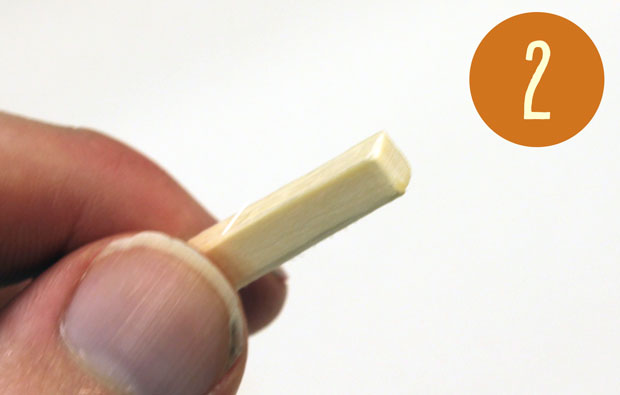Your disposable chopstick will act as a stylus for writing. Turn this stylus around so you are writing with the square end.
3.Let’s start with 1. Hold the stylus with the square end pointing away from you. Push one corner of the stylus into the clay and then pull it out to make a triangular dint, pointing towards you. This symbol is the Sumerian number 1.
4.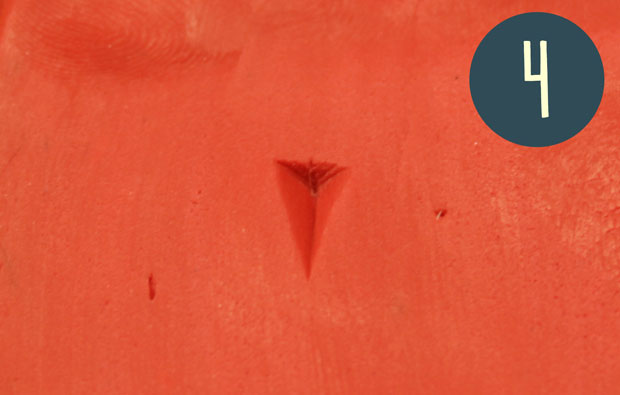Don’t worry if your dent looks a bit weird! Try writing several more 1s. Keep going until your 1s start to look the same. Then you can erase them all and start writing bigger numbers.
5.A 2 is just two 1 marks side by side, then and add another mark and you get 3. For 4, 5 and 6, start a second line of marks under the first. And for 7, 8 and 9 have a third line of marks under the second.

6.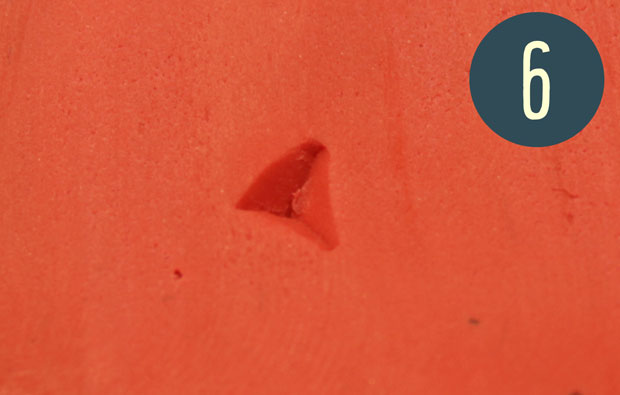To write 10, hold the stylus in your left hand, higher on the stylus than when you wrote 1. Hold the stylus across the clay and push the corner down. You will get a wider dint, pointing to the left.
7. You can combine these two symbols. For example, a 10 and then a 6 makes 16.
8.This is 35.

To write 20, simply write two 10s next to each other. Then 30 is three 10s. Next, 40 has a line of three 10s and a fourth 10 underneath, and 50 adds another 10 to the second row. Once again, you can add 1s to get all the way to 59.

9. The Sumerians didn’t have a symbol for 60. Instead, they simply wrote a 1 mark. (If you find this confusing, remember these numbers were invented before the first alphabet!)
10.How do you write 61? Write a 60 (which is just a 1 mark), leave a gap, and then write a 1 mark. You can write all the numbers up to 119 as a 60 and then something.

11. The number 120 is exactly the same as the number 2. Then 180 is the same as 3, and you can continue this pattern.
12. There’s more if you want to write really big numbers. The number 3599 is written as 59, then a gap, then 59. To write 3600, the Sumerians used the same symbol as they did for 60, a 1 mark.
13.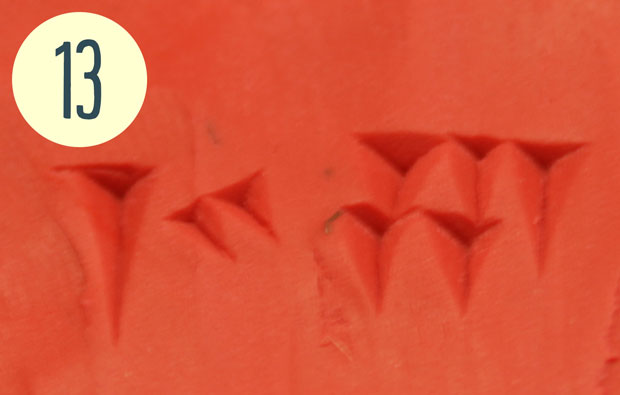Here’s a big number! this is 3605, written as a one, a zero and a five.

How do you write 3601? Here’s where the Sumerians used a special digit, kind of like our zero. First write 1, representing 3600. Then make two small dents, pointing at the bottom right of the clay. These symbols says that there are no 60s in this number. Finally, write a 1.

If you’re after more activities for kids, subscribe to Double Helix magazine!Categories:

Similar posts

1.Hi there! I’m trying to figure out how to write 2010. Would it be written as a 2, then 0 (the 2 indentions) followed by a 10 mark? Thanks in advance.

2.Hi me again for 2010. I think I figured it out. It would 33, then 0, then 33?

3.33 , 0 , 30. That’s what I meant

4.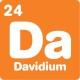Actually, you don’t need the zero to write 2010!
2010 = 33×60 +30×1, so you just write it as 30, 30.

in contrast, 30, 0, 30 = 33×3600 + 0x60 + 30×1 = 118 830

5.Great.
How could write 1979?
1 + 9 and 7 + 9?
TKS for help.

1.Hi Ricardo,
32 x 60 + 59 = 1979
So in Sumerian, it’s written as 32, 59
or two tens and two ones, then a gap, and then five tens and nine ones.

6.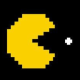How to write 256?:)

1.Hi Awab,
mathematically speaking, it’s four 60s, plus 16.
to write it, it’s four ones, a small gap, then a ten and six ones.
If you want a tattoo of it, I’d recommend reaching out to a university or museum that has a large Sumerian collections. There are a few bits and pieces that I glossed over in this activity, and it would be great to get a real expert to help you get it looking great.

7.Thank you,i will try to reach out to our local museum they have some,but i dont know if ill find someone who might help me there,if none ,university it is.